# Find all possible combinations of K numbers from 1 to n

Key takeaway: An excellent problem to understand the concept of problem-solving using backtracking and combinatorics. We can use similar ideas to solve other interview problems.

### Let’s understand the problem

Given two numbers n and K and you have to find all possible combinations of K numbers from 1 to n.

Example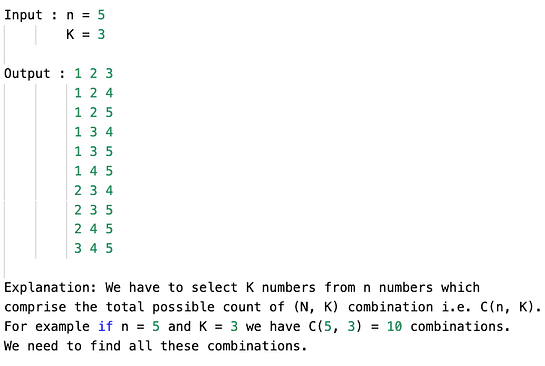#### Discussed solution approaches

• Inclusion and exclusion of every element
• Fix elements and recur for creating a combination of K numbers
• Recursive backtracking: DFS approach

### Inclusion and exclusion of every element

Solution Idea

We use the idea similar to the subset sum problem for creating possible combinations of K numbers from n numbers: we select each number from 1 to n and recur for two possible cases:

• The selected number is the part of the solution or included in the set
• The selected number is not part of the solution or not included in the set

Suppose we have n = 4 and K = 2. The following tree diagram explains the generation of all combinations of size 2.

• We are going left if we are including the number and going right if we are not including the number.
• At each level, we are including or excluding one number at a time.
• We have a combination of size 2 at each leaf node.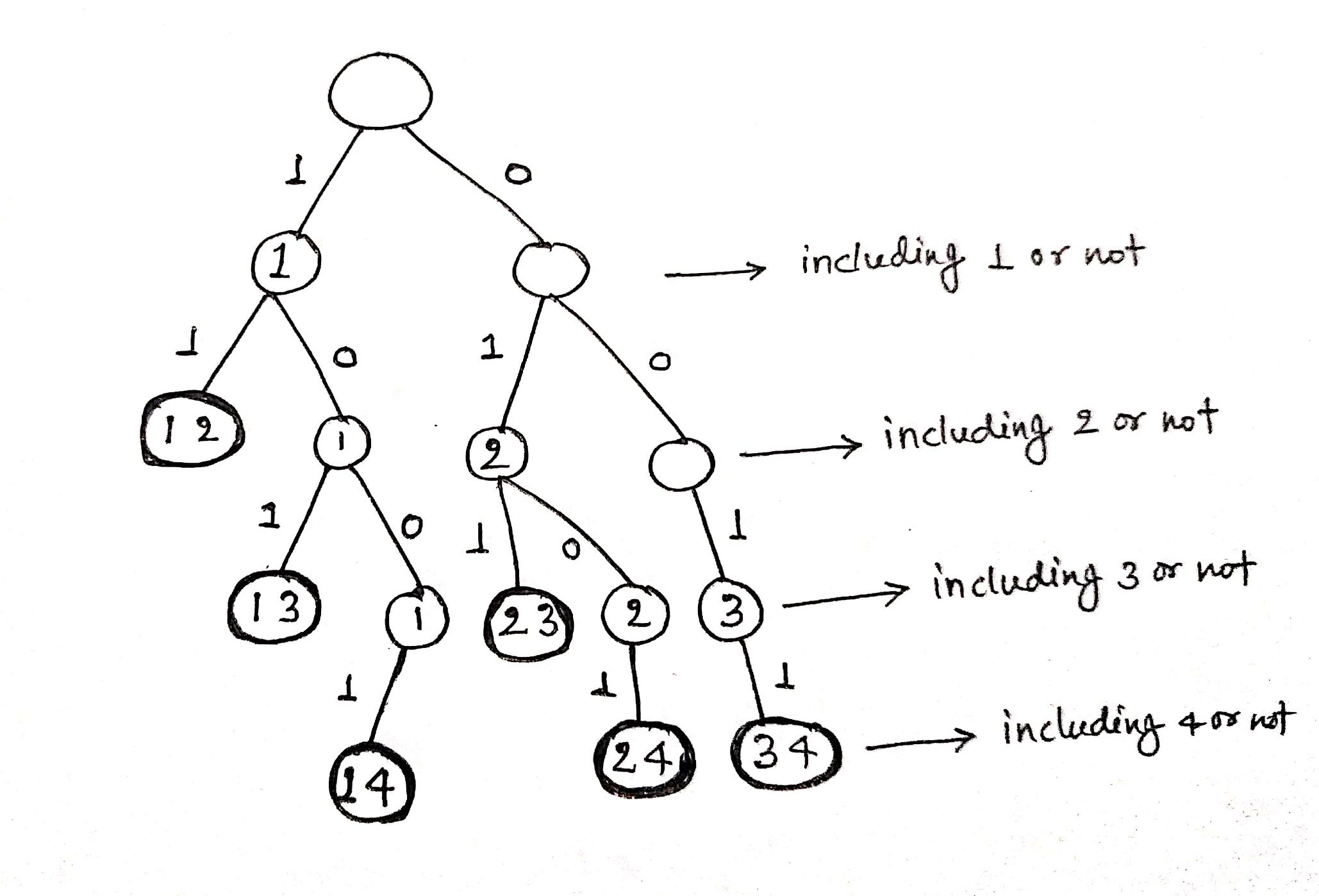To implement this, All we need to do is to consider both cases and recursively creates all possible combinations. Note: The idea of this approach comes from pascal’s Identity i.e: C(n, K) = C( n-1, K) + C(n-1, K-1) (Think!).

Suppose we use the function findKCombination(int K, int n), which returns output in a 2-D array. Inside the function, we declare two temporary arrays to store all outputs one by one:

• 1-D array temp[] to store any combination of K numbers.
• The 2-D array output[][] to store all possible combinations of K numbers

Now we use a helper function kCombination(int output[][], int temp[], int index, int n, int i, int K) to generate and store all K combinations in the output array using temp array. Here we also use a parameter index for excluding or including the current element in the combination and a parameter i for tracking the current element of the combination. Note: Our initial call is kCombination(output, temp, 0, n, 0, K).

Solution Pseudocode C++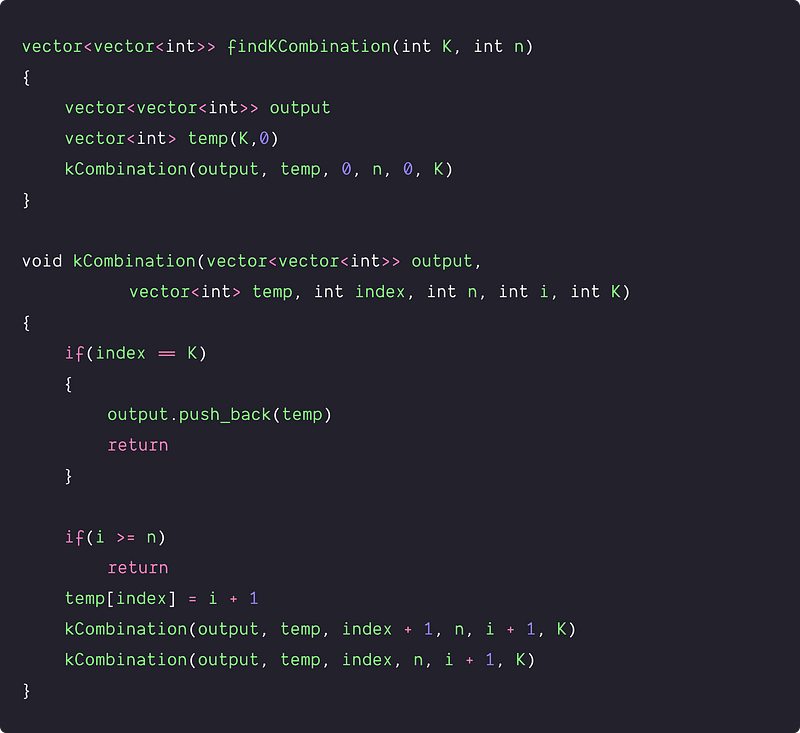Time and space complexity analysis

Here for each element, there are two possibilities i.e; whether the element will be selected or not. This creates two cases for each element and we are going to iterate for all n elements. So, the overall time complexity will be O(2^n). (Think!)

Critical ideas to think!

• Why would be the space complexity?
• How to handle duplicates in the above solution?
• How can we improve the time complexity?

### Fix elements and recur for creating a combination of K numbers

Solution Idea

The idea is to generate a combination tree where we fix each number from 1 to n and recursively build the combination of K numbers. Suppose we have n = 5 and K = 3.

• We first fix number 1 and recursively generate the all unique combination of size 3 starting with number 1 i.e. {1,2,3}, {1,2,4}, {1,2,5}, {1,3,4}, {1,4,5}.
• Then we first fix number 2 and recursively generate the all unique combination of size 3 starting with number 2 i.e. {2,3,4}, {2,3,5} and {2,4,5}.
• Then we first fix number 3 and recursively generate the all unique combination of size 3 starting with number 3 i.e. {3,4, 5}.
• There would be no unique combination of size 3 starting from 4 and 5. (Think!)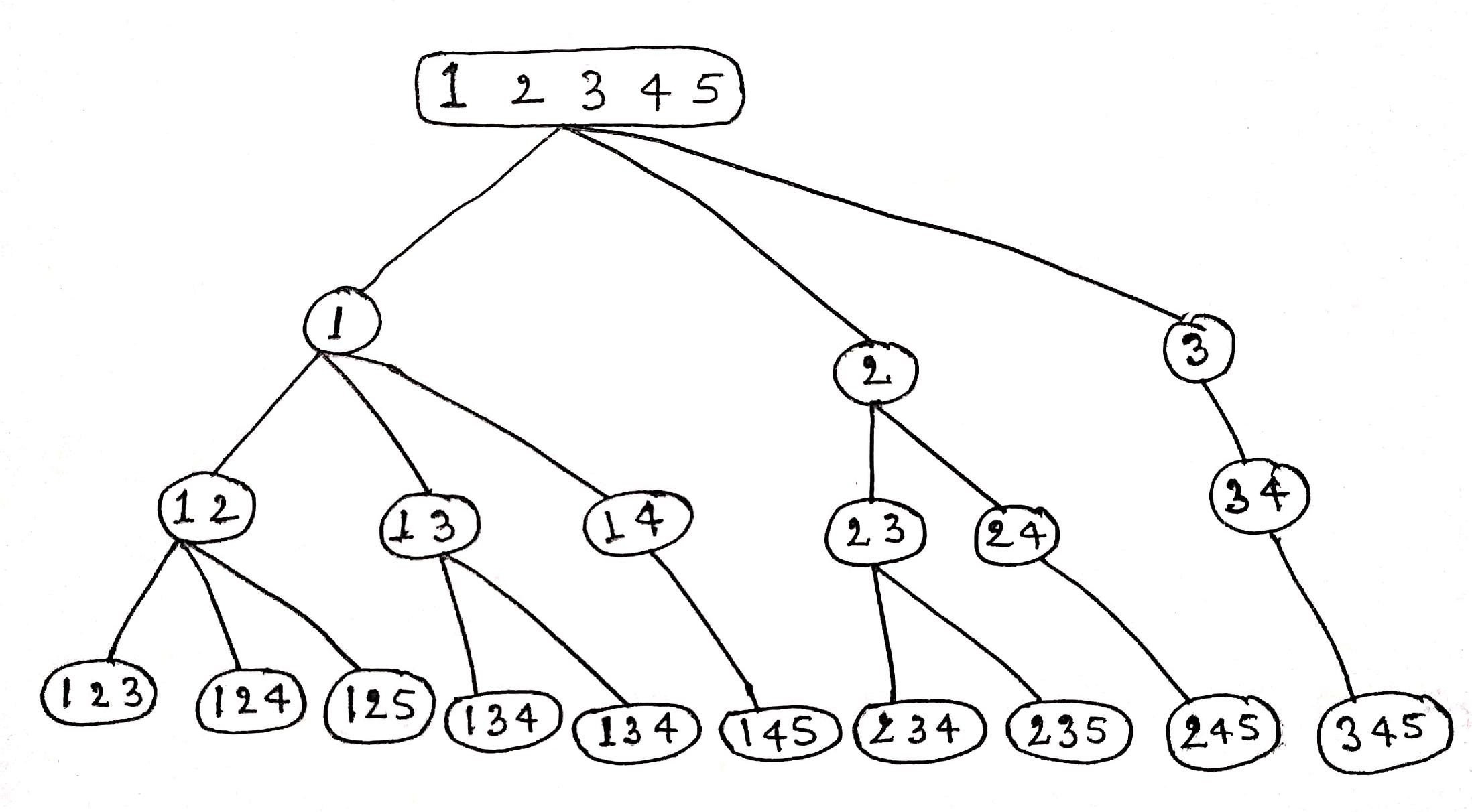To implement this:

• We use a temporary array temp[] to stores all outputs one by one.
• We start from the first index in temp[] and one by one fix elements at this index and recur for the remaining indexes.
• When the number of elements in temp[] becomes equal to K (size of a combination), recursion stop and we print temp[].

Solution Pseudocode C++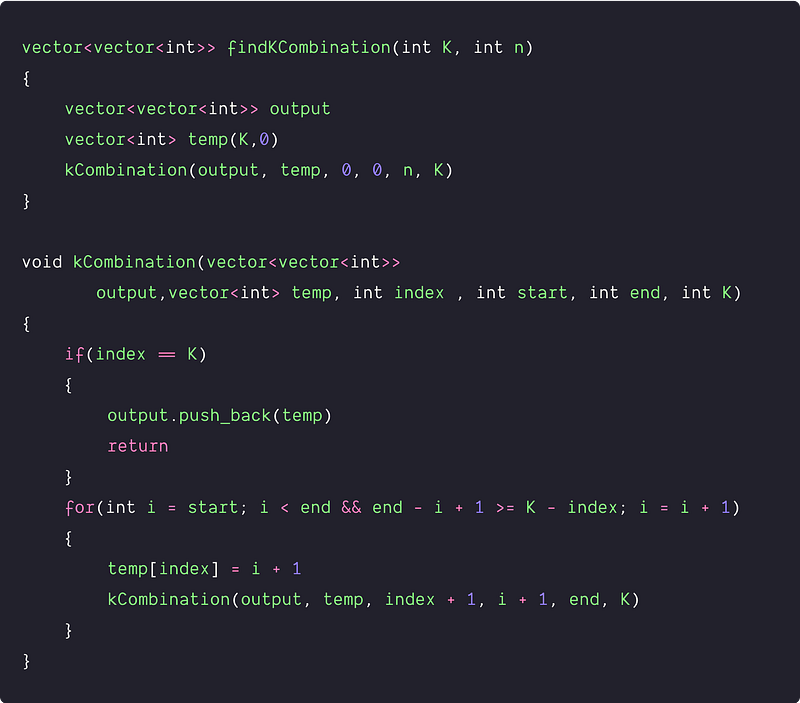Time and space complexity analysis

The loop in the algorithm will run C(n, K) times as this is the possible number of combinations and in each iteration, Elements can get selected in the range of (n-K) as K elements are already selected and we just replacing elements which are already occupied. So, the overall time complexity will be O(C(n, K) * (n-K)).

Space complexity = O(K* C(n, K)) (as we have total C(n, K) possible answer each having a size of K)

Critical ideas to think!

• Explain the derivation of space and time complexity?
• Why are we checking the condition end-i+1 ≥ k - ind inside the loop?
• Draw the recursion tree for the above code.
• Can we use this idea to solve other similar problems?
• is there a different way to implement the above solution?
• What are the modifications in the algorithm in case of duplicate numbers?
• can we improve the time complexity further?

### Recursive backtracking: DFS approach

Solution Idea

In this approach, we are using the power of DFS to recursively iterate through the range to generate all possible combinations. The iteration steps of the DFS approach are similar to the second approach we discussed above.

Here we iterate until we get a set consisting of K elements and store that subset in our resultant vector and then we backtrack and remove the previous element inserted in our temporary vector and consider further elements from the range which are not considered. This way all combinations are generated.

Solution Pseudocode C++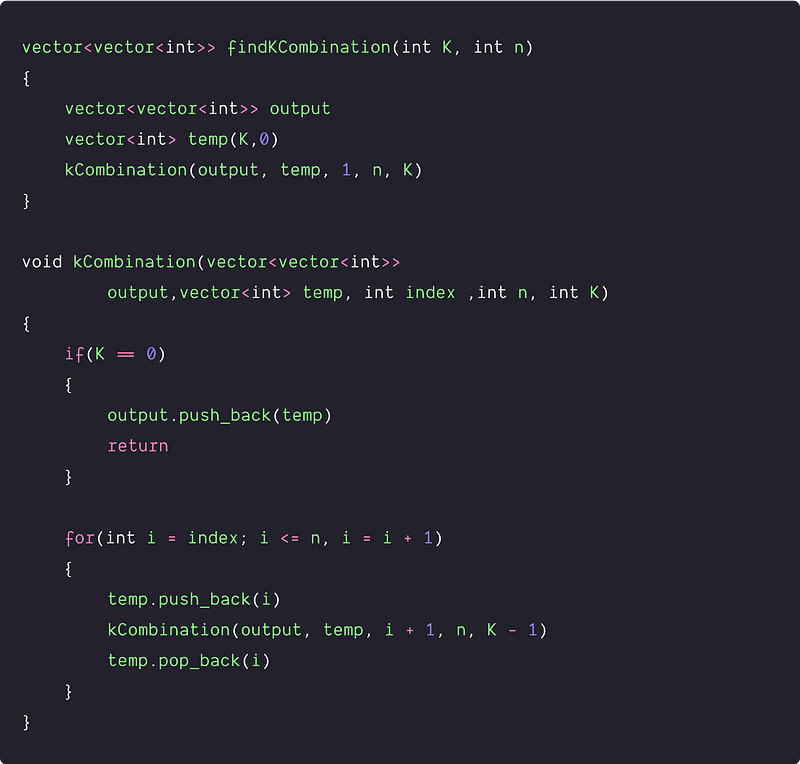Time and space complexity analysis

for loop can run for a maximum of n times with each backtrack with the maximum iteration of NcK times. i.e: overall time complexity will be O(N* C(n, K)).

Space Complexity = O(k* C(n, K))(as we have total C(n, K) possible answer each having a size of k )

Critical ideas to think!

• Explain the space and time complexity?
• Explore the best, average, and worst-case scenarios?
• The number of edge cases needs to be handled?
• what are the modifications in the algorithm in case of duplicate numbers?

### Suggested problems to practice

Enjoy learning, Enjoy problem-solving, Enjoy algorithms!

Get well-designed application and interview centirc content on ds-algorithms, machine learning, system design and oops. Content will be delivered weekly.